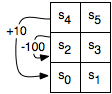Practice Exercise 11.A
Q-learning

## 2: Learning Goals

• Use the Q-learning algorithm to generate the table Q[S,A].
• Use a strategy which makes a trade off between exploration and exploitation.
• Modify parameters to maximize the performance of Q-learning agents.

## 3: Directed Questions

1. Given a set of states S, a set of actions A, and an experience ⟨s,a,r,s'⟩, what is the time complexity to update the value of Q(s,a) using Q-learning? [solution]

2. Consider the following Q[S,A] table:

State 1 State 2 1.5 2.5 4 3

Assume that α=0.1, and γ=0.5. After the experience ⟨1,1,5,2⟩, which value of the table gets updated and what is its new value? [solution]

3. What is one potential problem with a Q-learning agent that always chooses the action which maximizes the Q-value? [solution]

4. Describe two ways to force a Q-learning agent to explore. [solution]

## 4: Exercise: David's Tiny Game

Consider the tiny reinforcement learning problem shown in the following figure:This problem is also discussed in Section 11.3 of the textbook. There are six states the agent could be in, labeled as s0,...,s5. The agent has four actions: UpC, Up, Left, Right. That is all the agent knows before it starts. It does not know how the states are configured, what the actions do, or how rewards are earned. Suppose the actions work as follows:
upC
(for "up carefully") The agent goes up, except in states s4 and s5, where the agent stays still, and has a reward of -1.
right
The agent moves to the right in states s0,s2,s4 with a reward of 0 and stays still in the other states, with a reward of -1.
left
The agent moves one state to the left in states s1,s3,s5. In state s0, it stays in state s0 and has a reward of -1. In state s2, it has a reward of -100 and stays in state s2. In state s4, it gets a reward of 10 and moves to state s0.
up
With a probability of 0.8 it acts like upC, except the reward is 0. With probability 0.1 it acts as a left, and with probability 0.1 it acts as right.
The following applet can be used to simulate Q-learning for this problem:

Visit this page for instructions on how to use the applet. Consider the following parameter settings:
1. α=0.1, γ=0.5, Greedy Exploit = 80%, and initial Q-value = 0.
2. α=0.1, γ=0.5, Greedy Exploit = 80%, and initial Q-value = 20.
3. α=0.1, γ=0.5, Greedy Exploit = 100%, and initial Q-value = 0.
4. α=0.1, γ=0.5, Greedy Exploit = 100%, and initial Q-value = 20.
5. α=0.1, γ=0.1, Greedy Exploit = 80%, and initial Q-value = 0.
6. α=0.9, γ=0.5, Greedy Exploit = 80%, and initial Q-value = 0.
Run an experiment for each of these parameter settings and record the total reward received. [solution] Use a step size of 1,000,000. Manually move the robot to the bottom left square and press the reset button before each experiment. Keep in mind that since there is randomization involved, there will be some variation when running the same experiment multiple times.
• Compare the results of the first and second experiments to the third and fourth experiments. Changing the initial Q-value should have a much larger effect on one of these experimental pairs. Explain why these parameter settings cause this result. [solution]

• Compare the results of the first experiment to the third experiment. One should do significantly better than the other. Explain why the change in the Greedy Exploit parameter causes this result. [solution]

• Compare the results of the first experiment to the fourth experiment. One should do significantly better than the other. Explain why the change in the Greedy Exploit and initial Q-value parameters causes this result. [solution]

• Compare the results of the first experiment to the fifth experiment. One should do significantly better than the other. Explain why the change in the γ parameter causes this result. [solution]

• Compare the results of the first experiment to the sixth experiment. One should do significantly better than the other. Explain why the change in the α parameter causes this result. [solution]

## 5: Learning Goals Revisited

• Use the Q-learning algorithm to generate the table Q[S,A].
• Use a strategy which makes a trade off between exploration and exploitation.
• Modify parameters to maximize the performance of Q-learning agents.